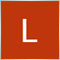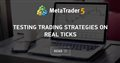# EA open position above the chart133

Hi guys, I build a simple EA that open a position according to EMA crossover. But when I backtest it I found an odd open position above the candle like on the image. This kind open position happened more than once and I don't know why it behave like that. Here's my EMA crossover code.
```int EMACrossing(int shift = 0)
{
int result = 0;
double EMAFastCurr = iMA(Symbol(),PERIOD_CURRENT,9,0,MODE_EMA,PRICE_CLOSE,shift);
double EMASlowCurr = iMA(Symbol(),PERIOD_CURRENT,21,0,MODE_EMA,PRICE_CLOSE,shift);
double EMAFastPrev = iMA(Symbol(),PERIOD_CURRENT,9,0,MODE_EMA,PRICE_CLOSE,shift+1);
double EMASlowPrev = iMA(Symbol(),PERIOD_CURRENT,21,0,MODE_EMA,PRICE_CLOSE,shift+1);
if(EMAFastPrev<EMASlowPrev && EMAFastCurr>EMASlowPrev)
result=1;
if(EMAFastPrev>EMASlowPrev && EMAFastCurr<EMASlowCurr)
result=2;
return(result);
}```

and here's my open position code.

```if(EMACrossing() == 1 && Volume==1)
{
double stopLossPrice = Ask - trailingStop*Point();
Print("Order ticket: ",OrderTicket());
}
if(EMACrossing() == 2 && Volume==1)
{
double stopLossPrice = Bid + trailingStop*Point();
orderId = OrderSend(Symbol(),OP_SELL,lotSize,Bid,0,stopLossPrice,0,NULL,magicNumber1);
Print("Order ticket: ",OrderTicket());
}```

anyone knows how this is happened?Testing trading strategies on real ticks
• www.mql5.com
The article provides the results of testing a simple trading strategy in three modes: " 1 minute OHLC " using only Open, High, Low and Close prices of minute bars; detailed modeling in " Every tick " mode, as well as the most accurate " Every tick based on real ticks " mode applying actual historical data. Comparing the results allows us to...
Files:
Capture.JPG 55 kb22306

Luandre Ezra I found an odd open position above the candle like on the image.

1. Your buy order's TP/SL (or Sell Stop's/Sell Limit's entry) are triggered when the Bid / OrderClosePrice reaches it. Using the Ask±n, makes your SL shorter and your TP longer, by the spread. Don't you want the specified amount used in either direction?

MODE_SPREAD (Paul) - MQL4 programming forum - Page 3 #25

3. The charts show Bid prices only. Turn on the Ask line to see how big the spread is (Tools → Options (control+O) → charts → Show ask line.)
Most brokers with variable spread widen considerably at end of day (5 PM ET) ± 30 minutes. My GBPJPY (OANDA) shows average spread = 26 points, but average maximum spread = 134.133

William Roeder:
1. Your buy order's TP/SL (or Sell Stop's/Sell Limit's entry) are triggered when the Bid / OrderClosePrice reaches it. Using the Ask±n, makes your SL shorter and your TP longer, by the spread. Don't you want the specified amount used in either direction?

Hi William, what do you mean by the specified amount? I used the Ask as the base line and add the stop loss which is 5 pips to trail it, is this not specified?22306

1. Luandre Ezra  what do you mean by the specified amount? I used the Ask as the base line and add the stop loss which is 5 pips to trail it, is this not specified?

You added 5 pips. Is that a specific amount?

2. `double stopLossPrice = Bid + trailingStop*Point();`
3. `if(EMACrossing() == 2 && Volume==1)`